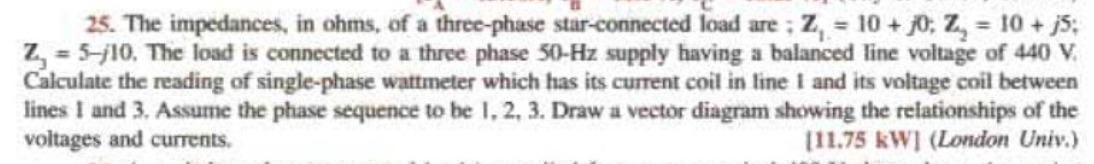Home / Expert Answers / Electrical Engineering / solve-it-handwritten-amp-correct-ans-is-mention-nbsp-show-step-39-s-nbsp-if-found-that-you-c-pa425

# (Solved): Solve it handwritten & Correct Ans is Mention   Show Step's.   If Found that You C ...

Solve it handwritten & Correct Ans is Mention

Show Step's.

If Found that You Copy From ai then dislike staright Forward ? & maybe Termination of your I'd.

Ai is Providing Wrong Answers

25. The impedances, in ohms, of a three-phase star-eonnected load are; $$\mathbf{Z}_{1}=10+j 0 ; \mathbf{Z}_{2}=10+j 5$$; $$\mathbf{Z}_{3}=5-j 10$$. The load is connected to a three phase $$50-\mathrm{Hz}$$ supply having a balanced line voltage of $$440 \mathrm{~V}$$. Calculate the reading of single-phase wattmeter which has its current coil in line 1 and its voltage coil between lines $$I$$ and 3. Assume the phase sequence to be $$1,2,3$$. Draw a vector diagram showing the relationships of the voltages and currents. [11.75 kW] (London Univ.)

We have an Answer from Expert